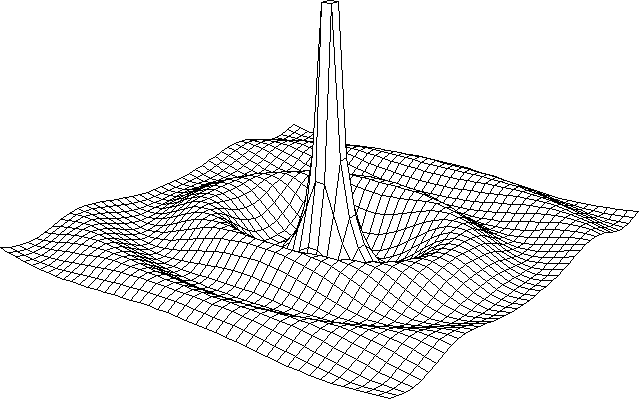# ICP 97

## Graph of two-parameter function

Write a program which will be able to display and rotate area described by function of two parameters z = f(x,y). The area should be displayed as a set of sections parallel with axis x and axis y (that means 3D form of graph).

After the start, program will ask you to type in a function. Function is defined by an expression which can contain variables x and y, numeric constants, parenthesis '(' and ')', operators '+' (add), '-' (subtract and unary minus), '*' (multiply), '/' (divide), '^' (power to) and functions `sqrt` (square root), `sin` (sine), `cos` (cosine), `tan` (tangent) a `ln` (natural logarithm).

After that, program will ask you for interval of drawing for each of variables x and y. After displaying area determined by the function, it will be possible to rotate the graph around z-axis (by keys left-arrow and right-arrow) and around x-axis (by keys up-arrow and down-arrow).

The program will automatically determine optimal size of the graph.Function: `4*cos(sqrt(2*x^2+y^2))/sqrt(x^2+y^2)` Interval for x and y: from -10 to 10